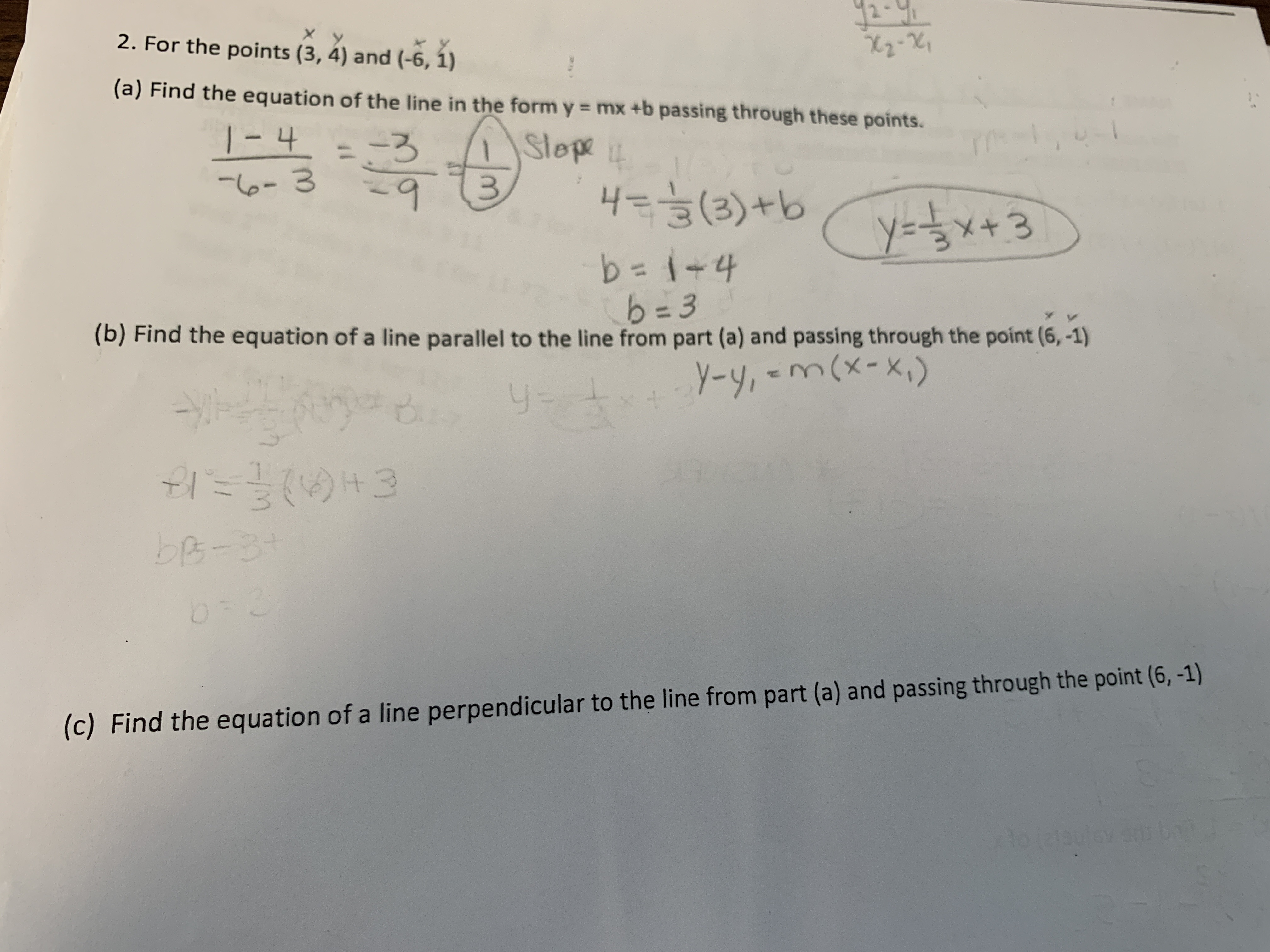# 2-2. For the points (3, 4) and (-6, 1)(a) Find the equation of the line in the form y = mx +b passing through these points.I 4Slepe0-3y-X+3b=14b=3(b)Find the equation of a line parallel to the line from part (a) and passing th rough the point (6,-1)y-y,-mx-"C.IbB-3(c) Find the equation of a line perpendicular to the line from part (a) and passing through the point (6, -1)xho leteule

Question
15 views

I need to know if A is correct and help with b and chelp_outlineImage Transcriptionclose2- 2. For the points (3, 4) and (-6, 1) (a) Find the equation of the line in the form y = mx +b passing through these points. I 4 Slepe 0-3 y- X+3 b=14 b=3 (b)Find the equation of a line parallel to the line from part (a) and passing th rough the point (6,-1) y-y,-mx- "C. I bB-3 (c) Find the equation of a line perpendicular to the line from part (a) and passing through the point (6, -1) xho leteule fullscreen
check_circle

Step 1

The points are (3,4) and (-6,1)

The slope of the line passing through the point is given by,

Step 2

Thus, the equation of line passing through the points (3,4) and (-6,1) and having slope m=1/3 is given by,

Step 3

The slope of parallel lines are always equal, So the slope of line parallel to the line in part a is given as ,m=1/...

### Want to see the full answer?

See Solution

#### Want to see this answer and more?

Solutions are written by subject experts who are available 24/7. Questions are typically answered within 1 hour.*

See Solution
*Response times may vary by subject and question.
Tagged in

### Other